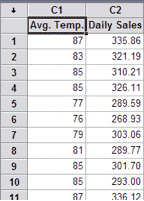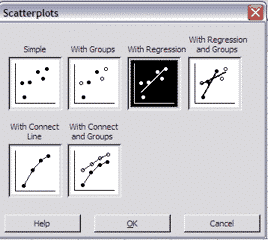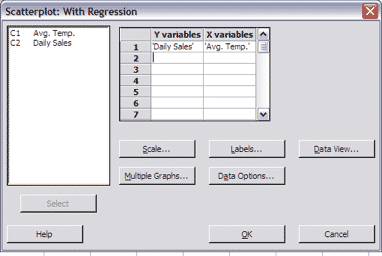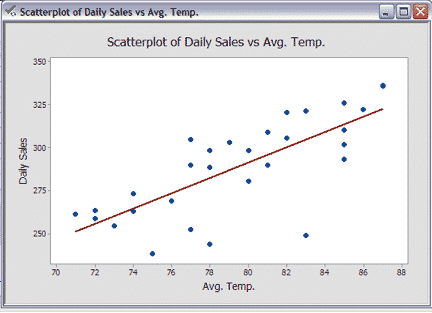## What’s a Scatter Plot?

A Scatter Plot is a chart that shows the relationship between two variables (if any).

A Scatter Plot is also known as an XY Plot since the variables are plotted on the X and Y axis. The strength of correlation on a Scatter Plot can be measured using a Correlation Coefficient.

## How to Create a Scatter Plot in Minitab

Download the GoLeanSixSigma.com Scatter Plot Data Set for Minitab here.

## 1. Open Scatter Plot Data Set:## 2. Go to Charts > Scatter Plot > With Regression:## 3. Click “OK”:## 4. Click “OK”: